# Numbers - math word problems

#### Number of problems found: 1462

• Sum of the seventeen numbersThe sum of the 17 different natural numbers is 154. Determine the sum of the two largest ones.
• Positive integersSeveral positive integers are written on the paper. Michaella only remembered that each number was half the sum of all the other numbers. How many numbers could be written on paper?
• Three numbers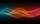The sum of the three numbers is 287. These numbers are in a ratio of 3: 7: 1/4 Define these numbers
• Two numbersThe sum of two numbers is 1. Identify this two numbers if you know that the half of first is equal to the third of second number.
• DigitsHow many five-digit numbers can be written from numbers 0.3,4, 5, 7 that is divided by 10 and if digits can be repeated.
• Insert into GPBetween numbers 5 and 640 insert as many numbers to form geometric progression so sum of the numbers you entered will be 630. How many numbers you must insert?
• Consecutive numbersSum of ten consecutive numbers is 105. Determine these numbers (write first and last).
• Common divisorsFind all common divisors of numbers 30 and 45.
• How many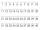How many double-digit numbers greater than 30 we can create from digits 0, 1, 2, 3, 4, 5? Numbers cannot be repeated in a two-digit number.
• AlgebrogramSolve algebrogram for sum of three numbers: BEK KEMR SOMR ________ HERCI
• Sum of odd numbersFind the sum of all odd integers from 13 to 781.
• Three-digitHow many three-digit natural numbers do not have the number 7?
• No. 215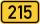From the number 215 we can create a four-digit number that among its numbers manually type any other digit. Thus, we created two four-digit numbers whose difference is 120. What two four-digit numbers that might be?
• Dd 2-digit numbersFind all odd 2-digit natural numbers compiled from digits 1; 3; 4; 6; 8 if the digits are not repeated.
• Progression-12, 60, -300,1500 need next 2 numbers of pattern
• Lcm of three numbersWhat is the Lcm of 120 15 and 5
• Two unknown numbersOne number is 2 less than a second number. Twice the second number is 14 less than 5 times the first. Find the two numbers.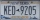A license plate has 3 letters followed by 4 numbers. Repeats are not allowed for the letters, but they are for the numbers. If they are issued at random, what is the probability that the 3 letters are in alphabetical order and the 3 numbers are consecutiv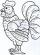Determine the quotient (q) and the remainder (r) from division numbers 100 and 8. Take the test of correctness.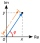Find the images of the following points under mappings: z=3-2j w=2zj+j-1